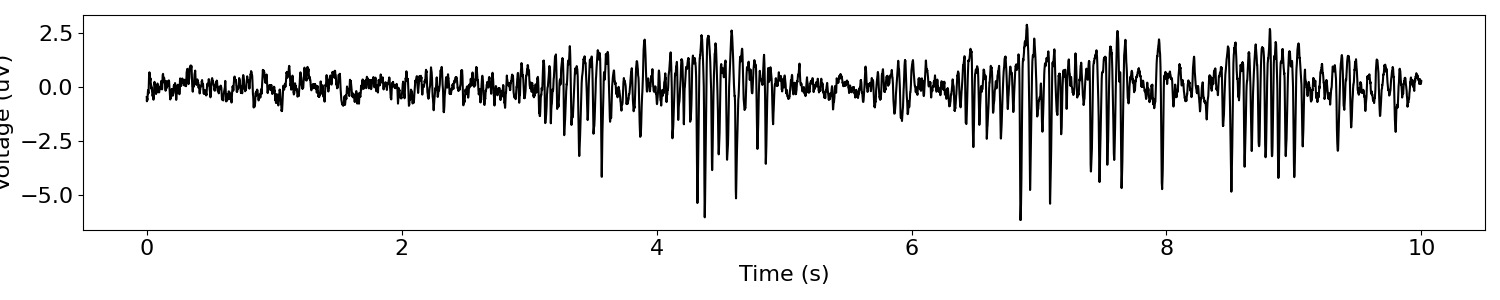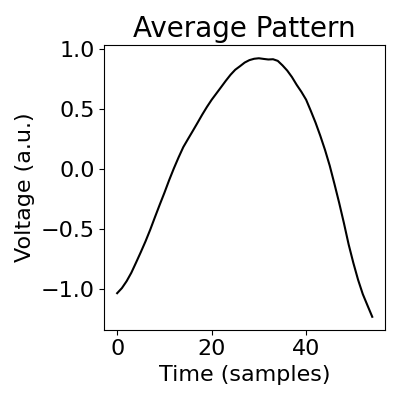# Sliding Window Matching¶

Find recurrent patterns in a neural signal using Sliding Window Matching.

This tutorial primarily covers the sliding_window_matching() function.

## Overview¶

This notebook shows how to use sliding window matching (SWM) for identifying recurring patterns in a neural signal, like the shape of an oscillatory waveform.

For more details on Sliding Window Matching see Gips et al., 2017, J Neuro Methods.

# Import the sliding window matching function
from neurodsp.rhythm import sliding_window_matching

from neurodsp.plts.rhythm import plot_swm_pattern
from neurodsp.plts.time_series import plot_time_series
from neurodsp.utils import set_random_seed, create_times

# Set random seed, for reproducibility
set_random_seed(0)


# Download, if needed, and load example data files

# Set sampling rate, and create a times vector for plotting
fs = 1000
times = create_times(len(sig)/fs, fs)

# Plot example signal
plot_time_series(times, sig)## Apply sliding window matching to neural signal¶

The loaded neural signal has a beta oscillation, that we can attempt to analyze with the sliding window matching approach.

We will define the window length to be about 1 cycle, which should roughly extract the waveform shape of the neural oscillation.

Sliding window matching can be applied with the sliding_window_matching() function.

# Define window length & minimum window spacing, both in seconds
win_len = .055
win_spacing = .2

# Apply the sliding window matching algorithm to the time series
avg_window, window_starts, costs = sliding_window_matching(sig, fs, win_len, win_spacing,
max_iterations=500)


You can plot the resulting pattern with plot_swm_pattern().

# Plot the discovered pattern
plot_swm_pattern(avg_window)Notice that the beta cycles have sharper troughs than peaks, and the average window is a beta cycle with a sharp trough.

One thing to explore is how these results change by changing the random seed.

Using more data and increasing the number of iterations helps the robustness of the algorithm.

Total running time of the script: ( 0 minutes 1.126 seconds)

Gallery generated by Sphinx-Gallery